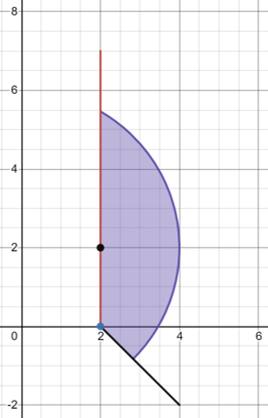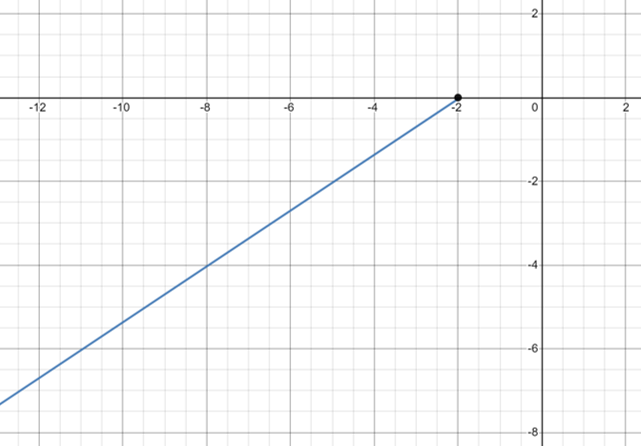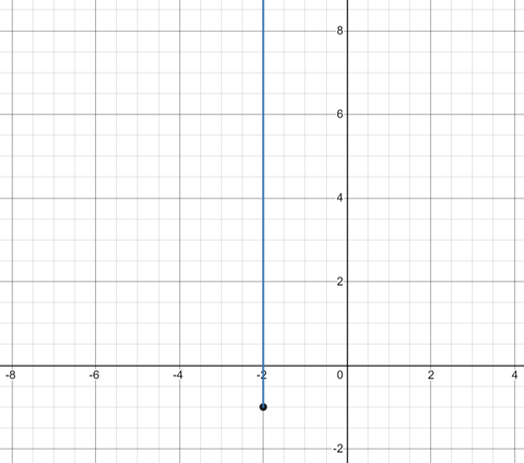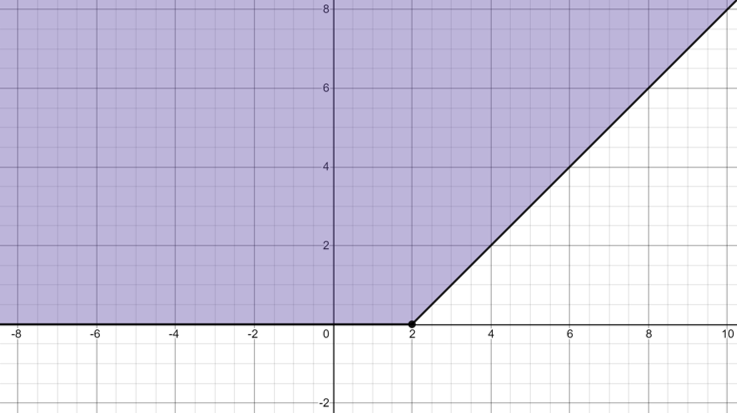# Complex Numbers Contd.

#### Question 1 - Part a

Complex Number z is represented by P(x,y) and
Complex Number w is represented by Q(u,v) in an argand diagram and
wz = 1

Show x =

 u u² + v²

And obtain an expression for y in terms of u and v

First we let w = u + ui and
z = x + yi as shown by there points on the argand diagram.
We also know that z = 1/w from the equation wz = 1

Using this information we can create the expression

x + yi =

 1 u + vi

 1 u + vi

=

 1 u + vi

x

 u - vi u - vi

=

 u - vi u² - v²i²

=

 u - vi u² + v²

x + yi =

 u - vi u² + v²

By equating the real and imaginary parts of the equation we find that

x =

 u u² + v²

and

y =

 -v u² + v²

#### Question 1 - Part b

Point P moves along the lin y = mx + 1
Show locus is a circle

Using the equations for y and x above we can represent this equation as:

 -v u² + v²

= m

 u u² + v²

+ 1

Mulitplying throughout by (u² + v²) gives us:
-v = mu + u² + v²
0 = mu + u² + v² + v

We can now use completing the square to get these values into terms:
0 = (u + m/2)² - (m/2)² + (v + 1/2)² - (1/2)²

Rearranging the equation so it fits that of a circle gives:
(u + m/2)² + (v + 1/2)² = (m/2)² + (1/2)²
(u + m/2)² + (v + 1/2)² = m²/4 + 1/4

This means that the:
Centre = (-m/2,-1/2)

m²/4 + 1/4

= (1/2) √

m² + 1

#### Question 2 - Part a

Complex Number z is represented by P(x,y) and
Complex Number w is represented by Q(u,v) in an argand diagram and
w = 1 + z²

And obtain an expression for u and v in terms of x and y

First we let w = u + ui and
z = x + yi as shown by there points on the argand diagram.
We also know that w = 1 + z²

Using this information we can create the expression

u + vi = (x + yi)² + 1
u + vi = x² + 2xyi - y² + 1

Equating the real and imaginary parts to find u and v we find:
u = x² - y² + 1
v = 2xy

#### Question 2 - Part b

Point P moves along the line y = 2x
Find the equation of the locus of Q in the form v = mu + c

Using the equations for y and x above we can represent this equation as simultaneous equations:

Equation 1:
v = 2xy
But y = 2x so:
v = 2x(2x)
v = 4x²
x² = v/4

Equation 2:
u = 1 + x² - y²
But y = 2x so:
u = 1 + x² - (2x)²
u = 1 - 3x²
Using Equation 1 for the value of x²
u = 1 - (3v)/4

Rearranging the equation so it becomes the form v = mu + c
4u = 1 - 3v
3v = -4u + 1
v = -(4/3)u + 1

#### Question 3 - Part a

Indicate clearly on an argand diagraam the points that satisfy:

 -π 6

≤ arg(z-2+i) ≤

 π 2

and |z-2i-2i| ≤ 4

First we need to look at the arg(z-2+i)
What arg tells us is the range in angles that the Point lies between and on
In this example we know that the Point lies between

 -π 6

and

 π 2

So now we know what the angles the point lies between and on we now need to find where those angles lie on the graph by looking inside the brackets of arg(z-2+i)
When plotting an argument we need to know that the coordinates are represented in the form
z - (Coordinates)
Rearranging our equation gives us:
z - (2 - i)
This means our angles stem from the point (2 - i)
[or P(2,-1)]

Now we need to look at the second equation
|z - 2 - 2i| ≤ 4
Looking at the left side of the equation we can find the point this represents using the form z - (Coordinates)
|z - (2+2i)|
This means this equation lies on the point Q(2,2)
Using our previous knowledge we know this means the magnitude of the point is less than or equal to 4
This means the point lies within 4 units of Q(2,2) creating a circle around the point

Combining these 2 requirements to find the points that satisfy both equations we find the graph:#### Question 3 - Part b

arg(z+2) =

 -2π 3

Rearranging the values inside the argument in the form
z - (Coordinates) gives us:
arg(z - [-2])
This mean the arg comes from point P(-2,0)

Now as there is only an equals in the equation this means that all potential points must lie upon this line and using the equation:

arg(z+2) =

 -2π 3

and knowing this line stems from the point P(-2,0) we can draw all the points on a graph like this:#### Question 3 - Part c

arg(z+2+i) =

 π 2

Rearranging the values inside the argument in the form
z - (Coordinates) gives us:
arg(z - [-2 - i])
This mean the arg comes from point P(-2,-1)

Now as there is only only an equals in the equation this means that all potential points must lie upon this line and using the equation:

arg(z+2+i) =

 π 2

and knowing this line stems from the point P(-2,-1) we can draw all the points on a graph like this:#### Question 3 - Part d

 π 3

< arg(z-2) < π

Rearranging the values inside the argument in the form
z - (Coordinates) gives us:
arg(z - )
This mean the arg comes from point P(2,0)

Now there is a greater than and less than sign in the equation this means that all potential points must lie between these 2 lines but not on them as they do not equal these values they only lie between them therefore using the equation:

 π 3

< arg(z-2) < π

and knowing this line stems from the point P(2,0) we can draw all the points on a graph like this:#### Question 4

Find the complex number z

2z* + iz = (1 + 2i)(2 - 3i)
We know that z = x + iy and z* = x - iy therefore:
2(x - iy) + i(x + iy) = (1 + 2i)(2 - 3i)
2x - 2iy + ix - y = 2 + 4i - 3i + 6
2x - 2iy + ix - y = i + 8

Equating the real and imaginary parts gives us:
Real: 2x -y = 8
Imaginary: -2y + x = 1

If we now multiply the real equation by 2 such that they both have -2y we get:
4x - 2y = 16

Taking the imaginary equation from the new real equation we get:
3x = 15
x = 5

Replacing x with 5 in the Imaginary Equation gives us:
-2y + 5 = 1
-2y = -4
y = 2

Therefore z = 5 + 2i